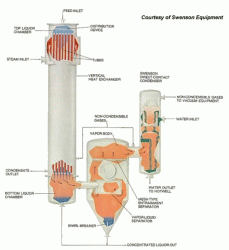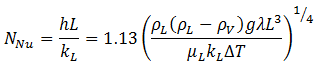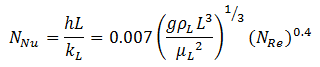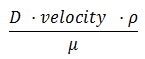Search Results

There were 1 results tagged with evaporators

By section

Sort by                Order
1. Falling Film Evaporators in the Food Industry

That orange juice that you had this morning sure tasted good didn't it?Â  Did you ever wonder how they get it concentrated into that little can?Â  Chances are the manufacturers used a falling film evaporator.Â

Falling film evaporators are especially popular in the food industry where many substances are heat sensitive.Â  {parse block="google_articles"}A thin film of the product to be concentrated trickles down inside the wall of the heat exchanging tubes. Â  Steam condenses on the outside of the tubes supplying the required energy to the inside of the tubes.Â  As the water from the process stream evaporates (inside the tubes) from the solids dissolved, the product become more and more concentrated.Â  The evaporators are designed such that a given flow of material can be concentrated to needed solids concentration by the time the stream exits the evaporator.Â  The two-phase product stream is usually run through a vapor-liquid separator after exiting the evaporator.Â  This separator allows the vapors to be drawn off the top and the concentrated liquid to exit the bottom (most falling film evaporators are run under vacuum conditions on the process side).

Understanding the Heat Transfer

The simple heat transfer balance for falling film evaporators is:Eq. (1)

where:Figure 1: Typical Falling Film Evaporator Configuration

Q = heat duty
U = overall heat transfer coefficient
A = heat transfer area
TS = temperature of condensing steam
T1 = boiling point of process liquid

The overall heat transfer coefficient consist of the steam side condensing coefficient (usually about 5700 W/m2 K), a metal wall with small resistance (depending on steam pressure, wall thickness), scale resistance on the process side, and a liquid film coefficient on the process side which will be extremely dependent on the viscosity of the process liquid over the concentration range.
Â Â Â

The steam side coefficient can be estimated as above or it can be calculated by the following equation for laminar flow:

ÂEq. (2)

Â andEq. (3)

for turbulent flow.Â  For the equations above,

ρL = liquid density (kg/m3)
ρV = vapor density (kg/m3)Â
g = 9.8066 m/s2
L = vertical height of tubes (M)
μL = liquid viscosity (Pa s)
μV = vapor viscosity (Pa s)
kL = liquid thermal conductivity (W/m K)
ΔT = Tsat-Twall (K)
λ = latent heat (J/kg)

All physical properties should be evaluated at the film temperature, Tf = (Tsat - Twall)/2 except for the latent heat which is evaluated at the saturation temperature.Â  The resistance due to scale formation cannot be predicted and will probably have to be estimated or compensated for by added a fouling coefficient or by added 5-10% to the calculated heat transfer area (sometimesÂ industry data is available for similar fluids to help estimate this value).Â Â

For the process fluid, the heat transfer coefficient can be calculated with the following expression:ÂEq. (4)

where:

b = 128 (SI units) or 39 (Imperial units)
NPr = Prandtl number =D = tube diameter
NRe = Reynolds number =ρ = density (subscript "L" for liquids, "V" for vapor)
μ = viscosity (subscript "L" for liquids, "V" for vapor)

Calculating pressure drops in falling film evaporators has been investigated since the late 1940's.Â  A universal equation is really not agreed upon.Â  Typically, a constant dependent on the percentage of vapor exiting the evaporator is used in a pressure drop relationship.Â  If your process fluid shares physical properties close to water, you may be able to accurately predict the pressure drop by using graphs and relations found in Perry's Chemical Engineers' Handbook.Â Â

• Oct 16 2012 01:34 PM
• by Chris Haslego